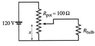# A light-dimmer switch with a potentiometer

• Asylum

## Homework Statement

Some light-dimmer switches use a variable resistor as shown in figure. The slide moves from position x = 0 to x = 1, and the resistance up to slide position x is proportional to x (the total resistance is Rpot = 100Ω at x = 1). What is the power expended in the light bulb if x = 0.5?

## Homework Equations

Rseries = R1 + R2 + ...
Rparallel = 1 / (1 / R1 + 1 / R2 + ...)
P = V2 / R = I2R

## The Attempt at a Solution

I tried first to obtain the total resistance, which is 50 + 1 / (1 / 50 + 1 / 205), and use this number to divide the voltage squared in order to get the power, but this is not correct. I don't know what I'm missing here. Any help will be appreciated.

#### Attachments

•P26_75.jpg
4.2 KB · Views: 614
Hi Asylum, Welcome to Physics Forums.

Were you given any information about the bulb itself? It's resistance or power rating?

Hi Asylum, Welcome to Physics Forums.

Were you given any information about the bulb itself? It's resistance or power rating?
Hello, I think the resistance for the bulb is 205Ω.

Okay, that would make it a 70 Watt bulb running at 120 V.

What you're looking to find is the power dissipated by the bulb resistance alone. To that end you'll need to find either the voltage across it, the current through it, or both.

Okay, that would make it a 70 Watt bulb running at 120 V.

What you're looking to find is the power dissipated by the bulb resistance alone. To that end you'll need to find either the voltage across it, the current through it, or both.
Thank you, I will try that now.